# The Pentagonal Cupola

The pentagonal cupola is one of the Johnson solids. It is bounded by 1 pentagon, 5 equilateral triangles, 5 squares, and 1 decagon, for a total of 12 faces, 25 edges, and 15 vertices. It is the 5th polyhedron in Norman Johnson's list, and bears the label J5.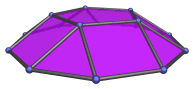A pentagonal cupola of edge length 2 has height √((10−2√5)/5), or approximately 1.05146, the same as the height of the pentagonal pyramid (J2). It is therefore a rather shallow shape, barely over half of its edge length. In 4D, this fact causes the segmentochoron decagonal_prism||pentagon, which has two pentagonal cupola cells, to be even shallower. This in turn has the consequence that a very large number of 10,n-duoprisms, up to n=20, can be augmented with this segmentochoron and remain convex, thus producing a large number of CRF augmented duoprisms.

A pentagonal cupola can be elongated by attaching a decagonal prism, forming an elongated pentagonal cupola (J20), or by attaching a decagonal antiprism, forming a gyroelongated pentagonal cupola (J24).

Pasting two pentagonal cupolae together in ortho orientation produces the pentagonal orthobicupola (J30). Doing so in gyro orientation produces the pentagonal gyrobicupola (J31).

Inserting a decagonal prism between the two cupolae produces, respectively, the elongated pentagonal orthobicupola (J38) and the elongated pentagonal gyrobicupola (J39).

Inserting a decagonal antiprism between the two cupolae produces instead the gyroelongated pentagonal bicupola (J46).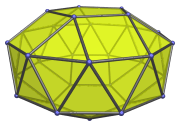Augmenting a pentagonal rotunda with a pentagonal cupola produces a pentagonal orthocupolarotunda (J32) or a pentagonal gyrocupolarotunda (J33), depending on its orientation with respect to the rotunda.

Inserting a decagonal prism between the rotunda and cupola produces the elongated pentagonal orthocupolarotunda (J40) and the elongated pentagonal gyrocupolarotunda (J41), respectively. Inserting a decagonal antiprism instead produces the gyroelongated pentagonal cupolarotunda (J47).

A truncated dodecahedron can be augmented with up to 3 pentagonal cupolae to form a series of augmented truncated dodecahedra:

## Projections

In order to be able to identify the pentagonal cupola in various projections of 4D objects, it is useful to know how it appears from various viewpoints. The following are some of the viewpoints that are commonly encountered:

Projection Envelope Description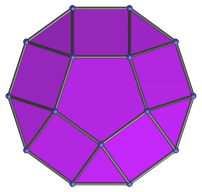Regular decagon

Parallel projection centered on pentagon.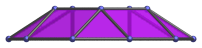Trapezium

Projection parallel to a pair of opposite decagon edges.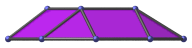Trapezium

Projection parallel to a pair of opposite decagon vertices.

## Coordinates

The pentagonal cupola may be described by the following coordinates:

• (0, ±2φ, 0, 0)
• (0, ±1, ±√(3+4φ), 0)
• (0, ±φ2, ±√(2+φ), 0)
• (0, √((10+2√5)/5), 2√((3−φ)/5))
• (0, ±φ, √((5−√5)/10), 2√((3−φ)/5))
• (0, ±1, −√((5+2√5)/5), 2√((3−φ)/5))

where φ=(1+√5)/2 is the Golden Ratio.

These coordinates produce a pentagonal cupola of edge length 2, resting on the XY plane and having its axis of symmetry aligned to the Z axis.

Alternative coordinates may be obtained by exploiting the fact that the pentagonal cupola occurs as part of the rhombicosidodecahedron, and thus may be cut from it. Cutting the rhombicosidodecahedron in this way yields the coordinates of the pentagonal cupola as:

 (±1, ±1, φ3) (0, ±φ2, φ+2) (φ2, ±φ, 2φ) (φ+2, 0, φ2) (φ, ±2φ, φ2) (2φ, ±φ2, φ) (φ3, ±1, 1)

These coordinates are algebraically nicer, in that they involve only simple expressions in terms of the Golden Ratio φ; however, the pentagonal cupola thus obtained is in an orientation unaligned with the coordinate axes.

Last updated 18 Jun 2019.